• WORK
• TOOLS
• TRAINING
• VIEWS
• CONTACT

Over-plotting in scatterplots is a problem in statistical graphs. It happens when one or more data point partially overlap or share the same location on the graph.

Stephen Few wrote a paper—which became part of his book Now You See It—in which he discussed six solutions to the problem of over-plotting:

· Reduce the size of data objects

· Remove fill color from data objects
· Change the shape of data objects
· Jitter data objects
· Make data objects transparent
· Reduce the amount of data

Here below you see 5 graphs. The first one illustrates the problem of over-plotting while the remaining graphs depict four of the six solutions proposed by Few.

Source: the graphs above are based on the Iris Data Set from Fisher 1936.

Sometimes the problem of overlap is a tough one and cannot be adequately resolved by applying the above solutions. This could be due to large datasets such as the scatterplot below showing the relationship between the total depth percentage and the length x of 53,940 round-cut diamonds.

# HOW TO CREATE A DENSITY SCATTERPLOT IN EXCEL

HOW TO CREATE A DENSITY SCATTERPLOT IN EXCEL

One solution to the overlap problem above is to encode the density of the overlap as shown in the density scatterplot below. The density scatterplot is a type of two-dimensional histogram showing the count of points in each region of the plot. In this this case the plotting region—the grey square—is divided into 40,000 cells (200*200) of equal size. We count the number of points that fall in each cell and portray the counts by varying the color intensity.

The data source for the density scatterplot is shown below. The table includes the total depth percentage and the length x for 53,940 round-cut diamonds.

1. On the worksheet, select columns B to GS. Right-click over the selected range and then, on the shortcut menu, click Column Width and set it to 0.21.
2. On the worksheet, select rows 6 to 205. Right-click over the selected range and then, on the shortcut menu, click Row Height and set it to 2. The worksheet should look like this:

Next, I need to define the bin width for the Depth percentage variable.

3. For that add the following formulas in the respective cells:
GW208 --> = 200
GV211 --> = MAX(GV217:GV54156)
GV212 --> = MIN(GV217:GV54156)
GV213 --> = GV211 - GV212
GV214 --> = GV213 / \$GW\$208
4. To define the bin width for the length x variable, select the range GV211:GV214 and press Ctrl + C to copy the cells into the clipboard. Next, select cell GW211 and press Ctrl + V to paste the content of the clipboard into the sheet. Add the labels and the worksheet should look like this:

Next, I need to define the boundaries of each bin for the Depth percentage variable.

5. For that add the following formulas in the respective cells:
B211 --> = GV212
C211 --> = B211 + \$GV\$214
6. Select cell C211 and press Ctrl + C to copy the cell into the clipboard. Next, select the range D211:GS211 and press Ctrl + V to paste the content of the clipboard into the sheet. The worksheet should look like this:

Next, I need to define the boundaries of each bin for the Length x variable.

7. For that add the following formulas in the respective cells:
GW205 --> = GW212
GW204 --> = GW205 + \$GW\$214
8. Select cell GW204 and press Ctrl + C to copy the cell into the clipboard. Next, select the range GW6:GW203 and press Ctrl + V to paste the content of the clipboard into the sheet. The worksheet should look like this:

9. Next I need to calculate the frequencies in each bin. for that enter the following formula in cell B205:
= COUNTIFS(\$GV\$217:\$GV\$54156,">=" & B\$211,\$GV\$217:\$GV\$54156,"<" & C\$211,\$GW\$217:\$GW\$54156,">=" & \$GW205,\$GW\$217:\$GW\$54156,"<" & \$GW204)
10. Select cell B205, press Ctrl + C to copy the formula into the clipboard, then select cells B7:GR205 and press Ctrl + V to paste the formulas from the clipboard.
11. Next, enter the following formula in cell GS205:
= COUNTIFS(\$GV\$217:\$GV\$54156,">=" & GS\$211,\$GW\$217:\$GW\$54156,">=" & \$GW205,\$GW\$217:\$GW\$54156,"<" & \$GW204)
12. Select cell GS205, press Ctrl + C to copy the formula into the clipboard, then select cells GS7:GS204 and press Ctrl + V to paste the formulas from the clipboard.
13. Next, enter the following formula in cell B6:
= COUNTIFS(\$GV\$217:\$GV\$54156,">=" & B\$211,\$GV\$217:\$GV\$54156,"<" & C\$211,\$GW\$217:\$GW\$54156,">=" & \$GW6)
14. Select cell B6, press Ctrl + C to copy the formula into the clipboard, then select cells C6:GR6 and press Ctrl + V to paste the formulas from the clipboard.
15. Next, enter the following formula in cell GS6:
= COUNTIFS(\$GV\$217:\$GV\$54156,">=" & GS\$211,\$GW\$217:\$GW\$54156,">=" & \$GW6)

16. Next, on the worksheet, select cells B6:GS205. Then, click on the Borders icon in the Font group under the Home tab. On the dropdown menu click More Borders.
17. In the Format Cells dialog box, on the Border tab, under Line, set the Style to the thick line (second before last) and the Color to grey. Then, under Presets, click the Outline button to indicate the border placement. Hence, I’ve defined a matrix with 200 rows and 200 columns. Click the Ok button to close the Format Cells dialog box.
18. Turn off the gridlines and the worksheet should look like this:

19. On the worksheet, select the range B6:GS205. Then, on the Home tab, in the Styles group, click the arrow next to Conditional Formatting, and then select Color Scales > More Rules.
20. In the New Formatting Rule dialog box, select Number from the Type dropdown list under Minimum, set the Value to 0 and change the Color to light yellow then change the Color of the Maximum to red as follows:

William Cleveland and Robert McGill—in their paper The Many Faces of Scatterplots—proposed the Sunflowers graph as another solution to the problem of overplotting. As you see in the graphs below, a single dot means one point. A dot with lines (petals) means many dots share that location. The number of petals reflect the number of dots around that specific location.

Views > Excel charts > How to create a density scatterplot in Excel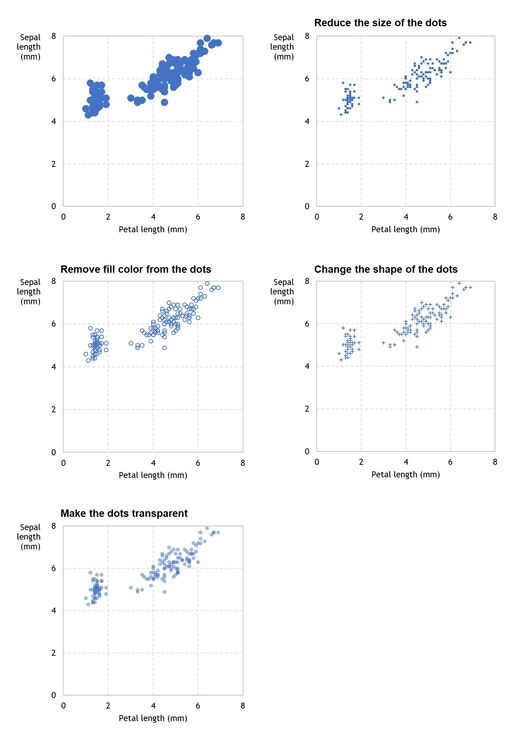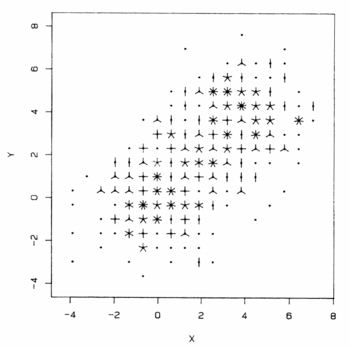Source: William Cleveland and Robert McGill, The Many Faces of Scatterplots 1984.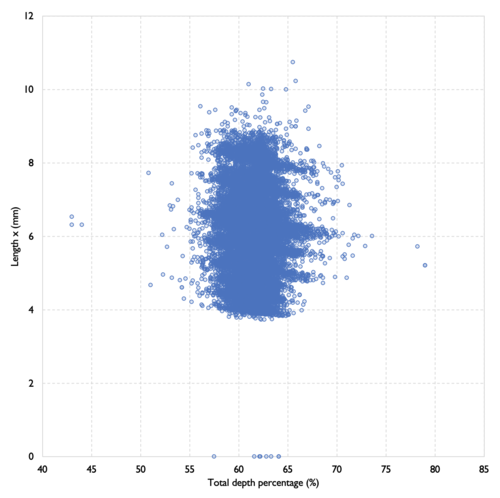Source: the graph above is based on the Diamond Data Set.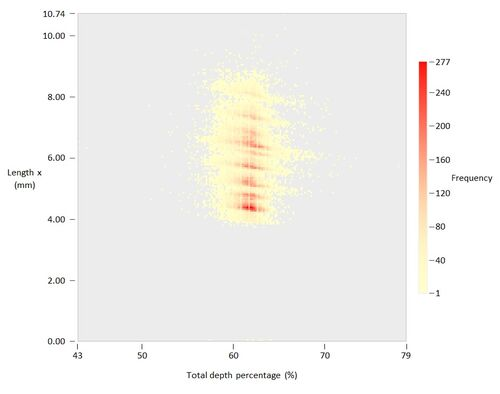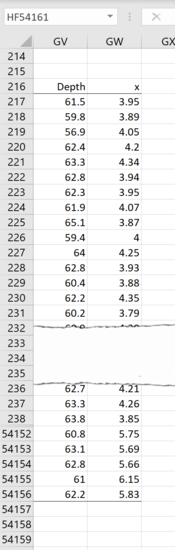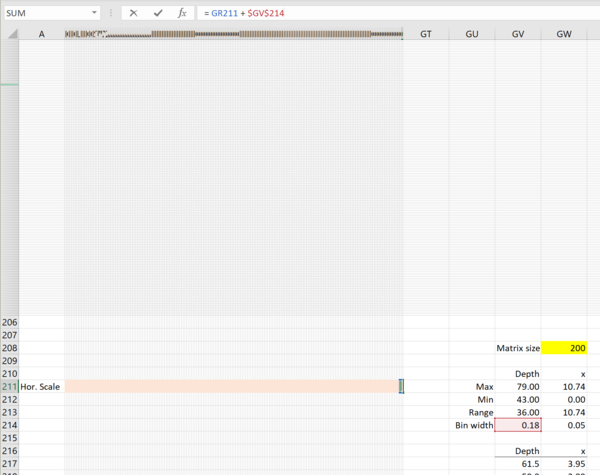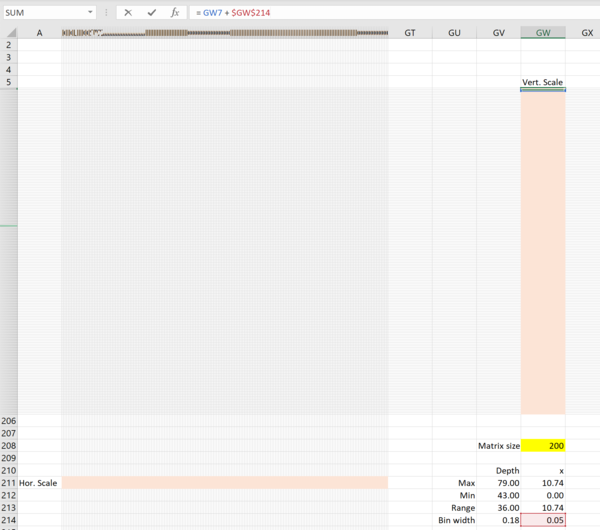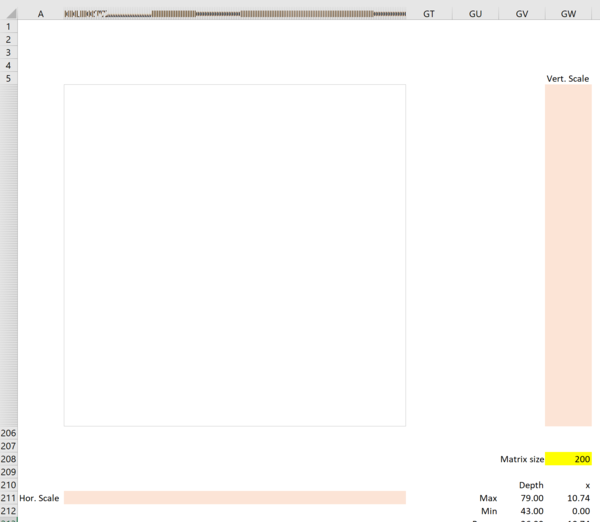25. Add the scale key using Shapes and the scale labels using text boxes.
26. Add title and sources and the chart should look like this:

20. Press the Ok button to close the New Formatting Rule dialog boxes. The worksheet should look like this:

21. While the range B6:GS205 is still selected, then on the Home tab, in the Styles group, click the arrow next to Conditional Formatting, and then select New Rule.
22. In the New Formatting Rule dialog box, click on the Use a formula to determine which cells to format option under Select a Rule Type.
22. In the input line Format values where this formula is true under Edit the Rule Description write the following formula:
= B6 = 0
as follows:

23. Click on the Format button in the Preview area. Next, in the Format Cells dialog box, under the Fill tab, click a color of your choice (say light grey) under Background Color.
24. Press the Ok button twice to close the Format Cells and New Formatting Rule dialog boxes. The worksheet should look like this: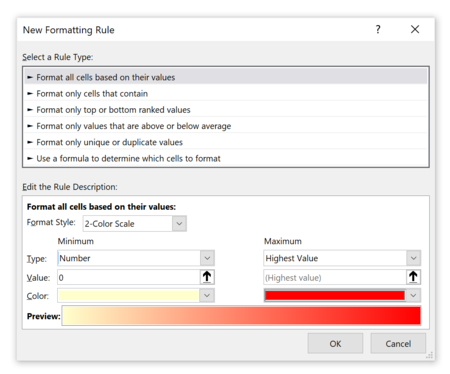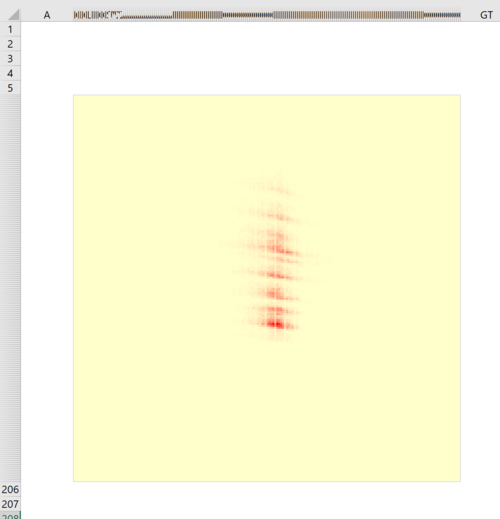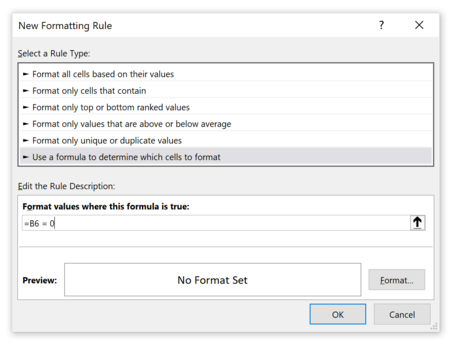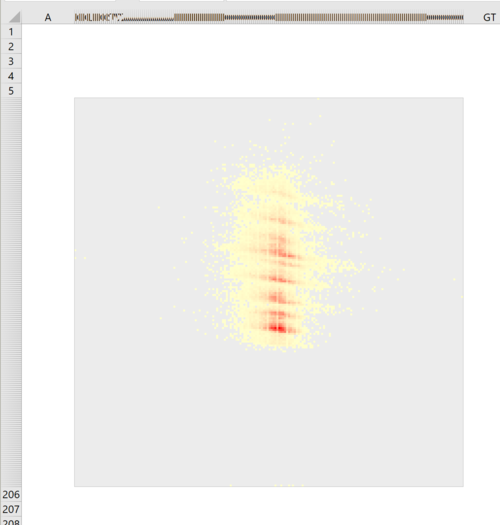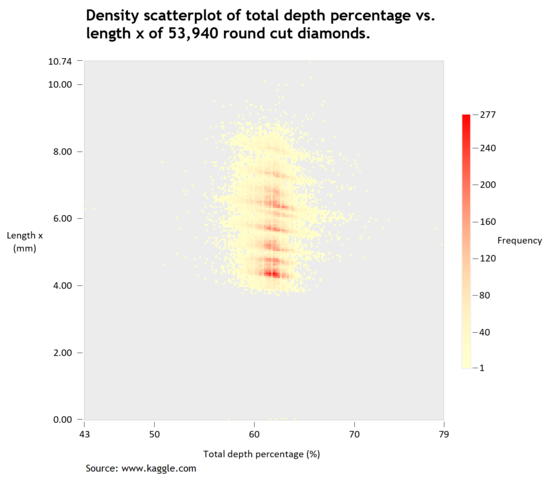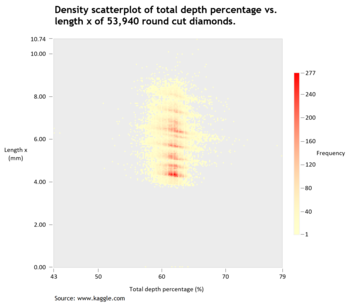Thank you for reading, and I hope you found this useful. If you have any questions, find me on Twitter and ask me anything.